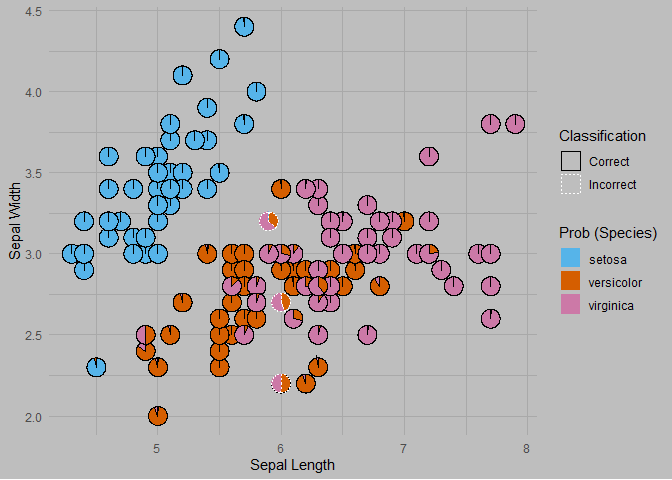# Multinomial Classification

This example presents the application of the PieGlyph package to show the predicted probabilities of different classes in a multinomial classification problem. This example could be useful for clustering problems which gives probabilities, e.g. mclust using model based clustering or ranger using random forests.

# install.packages('ranger')
library(ranger)
library(ggplot2)
library(PieGlyph)
library(dplyr)

We are using iris dataset which gives the measurements of the sepal length, sepal width, petal length and petal width for 50 flowers from each of Iris setosa, Iris versicolor, and Iris virginica

head(iris)
#>   Sepal.Length Sepal.Width Petal.Length Petal.Width Species
#> 1          5.1         3.5          1.4         0.2  setosa
#> 2          4.9         3.0          1.4         0.2  setosa
#> 3          4.7         3.2          1.3         0.2  setosa
#> 4          4.6         3.1          1.5         0.2  setosa
#> 5          5.0         3.6          1.4         0.2  setosa
#> 6          5.4         3.9          1.7         0.4  setosa

### Classification model

We use the random forest algorithm for classifying the samples into the three species according to the four measurements described above.

rf <- ranger(Species ~ Petal.Length + Petal.Width +
Sepal.Length + Sepal.Width,
data=iris, probability=TRUE)

We get the predicted probabilities of each sample belonging to a particular species.

preds <- as.data.frame(predict(rf, iris)\$predictions)
#>      setosa   versicolor virginica
#> 1 1.0000000 0.0000000000         0
#> 2 0.9976667 0.0023333333         0
#> 3 1.0000000 0.0000000000         0
#> 4 1.0000000 0.0000000000         0
#> 5 1.0000000 0.0000000000         0
#> 6 0.9997143 0.0002857143         0

Combine the predicted probabilities with the original data for plotting

plot_data <- cbind(iris, preds)
#>   Sepal.Length Sepal.Width Petal.Length Petal.Width Species    setosa
#> 1          5.1         3.5          1.4         0.2  setosa 1.0000000
#> 2          4.9         3.0          1.4         0.2  setosa 0.9976667
#> 3          4.7         3.2          1.3         0.2  setosa 1.0000000
#> 4          4.6         3.1          1.5         0.2  setosa 1.0000000
#> 5          5.0         3.6          1.4         0.2  setosa 1.0000000
#> 6          5.4         3.9          1.7         0.4  setosa 0.9997143
#>     versicolor virginica
#> 1 0.0000000000         0
#> 2 0.0023333333         0
#> 3 0.0000000000         0
#> 4 0.0000000000         0
#> 5 0.0000000000         0
#> 6 0.0002857143         0

Add a column indicating whether the sample was classified correctly or not

plot_data <- plot_data %>%
# Do operations on a row basis
rowwise() %>%
# Select the species with the highest predicted probability as the classified species
mutate(Predicted = colnames(.)[5 + which.max(c(setosa, versicolor, virginica))]) %>%
# Compare whether the selected species is same as the original
mutate('Classification' = ifelse(Species == Predicted, 'Correct', 'Incorrect')) %>%
ungroup()

### Create plot

The plot shows a scatterplot of the sepal width and sepal length for the samples in the iris dataset. The predicted probabilities of belonging to a particular species for each sample are shown by the pie-chart glyphs. The borders of the pie charts show whether or not the sample was classified correctly.

ggplot(data=plot_data,
aes(x=Sepal.Length, y=Sepal.Width))+
# Pies-charts showing predicted probabilities of the different species
# Using the pie-border to highlight if the same was classified correctly
geom_pie_glyph(aes(linetype = Classification,  colour = Classification),
slices = names(preds)) +
# Colours for sectors of the pie-chart
scale_fill_manual(values = c('#56B4E9', '#D55E00','#CC79A7'))+
# Labels for axes and legend
labs(y = 'Sepal Width', x = 'Sepal Length', fill = 'Prob (Species)')+
# Adjusting the borders colours and linetypes
scale_linetype_manual(values = c(1, 3))+
scale_colour_manual(values = c('black', 'white'))+
# Theme of the plot
theme_minimal()+
theme(panel.grid = element_line(colour = 'darkgrey'),
plot.background = element_rect(fill = 'grey', colour = NA))